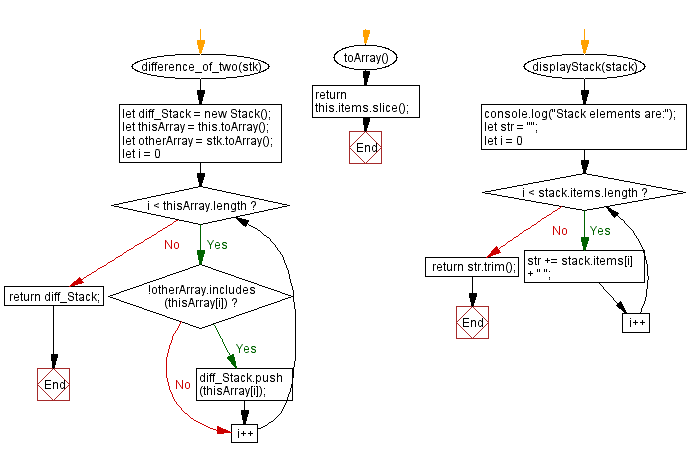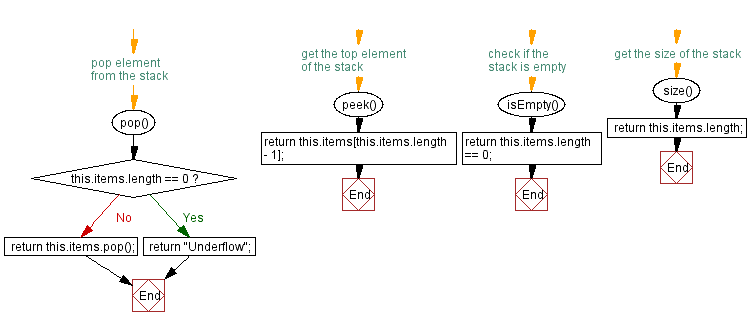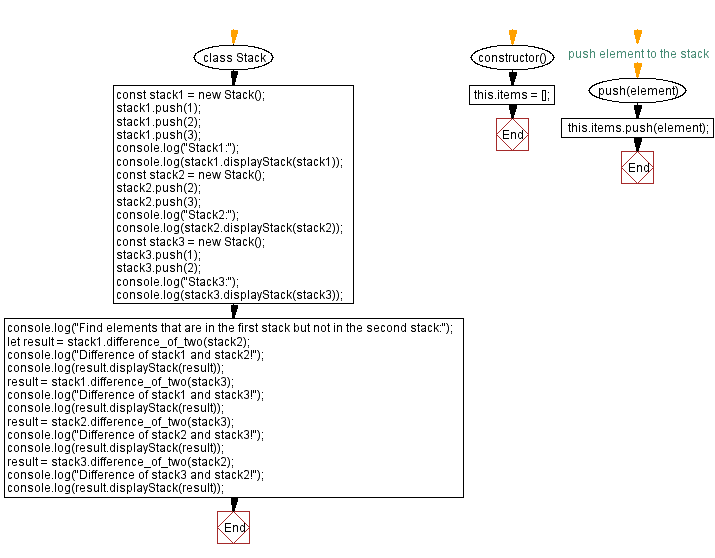# JavaScript Exercises: Stack elements in the first but not in the second

## JavaScript Stack: Exercise-29 with Solution

Write a JavaScript program that implements a stack and find elements that are in the first stack but not in the second stack.

Sample Solution:

JavaScript Code:

``````class Stack {
constructor() {
this.items = [];
}

// push element to the stack
push(element) {
this.items.push(element);
}

// pop element from the stack
pop() {
if (this.items.length == 0) {
return "Underflow";
}
return this.items.pop();
}

// get the top element of the stack
peek() {
return this.items[this.items.length - 1];
}

// check if the stack is empty
isEmpty() {
return this.items.length == 0;
}

// get the size of the stack
size() {
return this.items.length;
}

difference_of_two(stk) {
let diff_Stack = new Stack();
let thisArray = this.toArray();
let otherArray = stk.toArray();
for (let i = 0; i < thisArray.length; i++) {
if (!otherArray.includes(thisArray[i])) {
diff_Stack.push(thisArray[i]);
}
}
return diff_Stack;
}

toArray() {
return this.items.slice();
}

displayStack(stack) {
console.log("Stack elements are:");
let str = "";
for (let i = 0; i < stack.items.length; i++)
str += stack.items[i] + " ";
return str.trim();
}
}

const stack1 = new Stack();
stack1.push(1);
stack1.push(2);
stack1.push(3);
console.log("Stack1:");
console.log(stack1.displayStack(stack1));
const stack2 = new Stack();
stack2.push(2);
stack2.push(3);
console.log("Stack2:");
console.log(stack2.displayStack(stack2));
const stack3 = new Stack();
stack3.push(1);
stack3.push(2);
console.log("Stack3:");
console.log(stack3.displayStack(stack3));
console.log("Find elements that are in the first stack but not in the second stack:");
let result = stack1.difference_of_two(stack2);
console.log("Difference of stack1 and stack2!");
console.log(result.displayStack(result));
result = stack1.difference_of_two(stack3);
console.log("Difference of stack1 and stack3!");
console.log(result.displayStack(result));
result = stack2.difference_of_two(stack3);
console.log("Difference of stack2 and stack3!");
console.log(result.displayStack(result));
result = stack3.difference_of_two(stack2);
console.log("Difference of stack3 and stack2!");
console.log(result.displayStack(result));
```
```

Sample Output:

```"Stack1:"
"Stack elements are:"
"1 2 3"
"Stack2:"
"Stack elements are:"
"2 3"
"Stack3:"
"Stack elements are:"
"1 2"
"Find elements that are in the first stack but not in the second stack:"
"Difference of stack1 and stack2!"
"Stack elements are:"
"1"
"Difference of stack1 and stack3!"
"Stack elements are:"
"3"
"Difference of stack2 and stack3!"
"Stack elements are:"
"3"
"Difference of stack3 and stack2!"
"Stack elements are:"
"1"
```

Flowchart:Live Demo:

See the Pen javascript-stack-exercise-29 by w3resource (@w3resource) on CodePen.

Improve this sample solution and post your code through Disqus

Stack Previous: Find elements that are common in two stacks.
Stack Exercises Next: Elements from both stacks without duplicates.

What is the difficulty level of this exercise?

Test your Programming skills with w3resource's quiz.

﻿

## JavaScript: Tips of the Day

Creates an array of elements, ungrouping the elements in an array produced by zip and applying the provided function

Example:

```const tips_unzip = (arr, fn) =>
arr
.reduce(
(acc, val) => (val.forEach((v, i) => acc[i].push(v)), acc),
Array.from({
length: Math.max(...arr.map(x => x.length))
}).map(x => [])
)
.map(val => fn(...val));

console.log(tips_unzip([[2, 15, 200], [3, 25, 300]], (...args) => args.reduce((acc, v) => acc + v, 0)));
```

Output:

```[5, 40, 500]
```